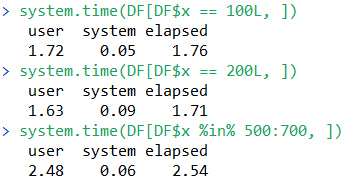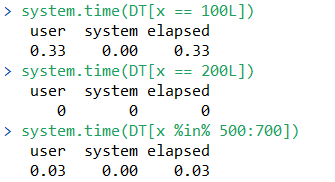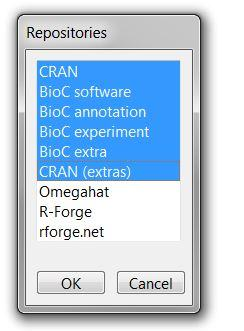# Need for Processing Speed: data.table

The first time I discovered data.table it felt like magic. I was waiting on a process that was projected to take the better part of an afternoon. In the meantime, I followed the data.table tutorial, rewrote my code using the data.table structure, and fully executed said code, all while the data.frame equivalent was wheezing along. In the last year, data.table has gotten even faster.

### data.table’s Automatic Indexing

For the uninitiated, data.table is a data structure that extends upon data.frame and streamlines the functionality for the age of “big data”. There are some syntactical differences, most of which are covered in this handy cheat sheet.

As of data.table package version 1.9.4 operations of the form DT[x == .] and DT[x %in% .] have been optimised to, whenever possible, build an index automatically on the first run, so that successive runs can make use of binary search for fast subsets instead of vector scans. Note that this query optimization is taken care of internally, and requires no user-end specification.

Let’s consider a toy example. Suppose we have a data.frame with column ‘x’ of length 10-million, whose values consist of integers randomly sampled between 1 and 10000. Further suppose we save said object as both a data.frame and a data.table.

``````DF = data.frame(x=sample(x = 1e4, size = 1e7, replace = TRUE), y = 1L)
DT = as.data.table(DF)
``````

Next, we perform simple operations on each. For example, “return all rows where x takes the value ‘100L’”. The use of ‘L’ indicates we are looking exclusively for integers, and gives some small gains in computation time.

For the data.frame, we have the following:For data.table, the first run will be fast, but much of the computation time is devoted to automatically building an index. Subsequent runs use the binary search with these indices, and are therefore even faster.The first run is dramatically faster than the data.frame equivalent, but after indexing, the second run takes virtually no time at all! However, note that as the time for the first data.table run includes time to create an index and perform a binary search, there may be instances that the first call of data.table may be slightly slower than a vector scan equivalent. Successive calls (after indexing) will be significantly faster for data.table, as evidenced above.

Additionally, further data.table query optimizations are in the works.

``````setRepositories()